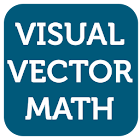Visual Vector Math

• SEARCH TYPE

All Android applications categories

All Android games categories# Visual Vector Math

by: 111 7.8

7.8 Users
rating

## Screenshots

Description

Visual Vector Math was created to provide a way to visualize vectors and the different operations and the effects of those operations.

The app has arithmetic ability to do addition, subtraction, multiplication, and division with vectors.

The app also has the ability to do vector operations:Vector cross product, dot(scalar) product, unit vector calculation, and magnitude calculation.

Tags: math vector , visual vector math , video test for the visual vector

## Users review

from 111 reviews

"Great"

7.8